# Statistics and meaning of name Matharu

Usage: 1% firstname, 99% surname.
Matharu first name was found 32 times in 6 different countries.
Surname Matharu is used at least 1593 times in at least 19 countries.Given namesAjit Matharu (19)Daljit Matharu (11)Davinder Matharu (10)Manjit Matharu (9)Kamaljit Matharu (8)Jaswinder Matharu (8)Balbir Matharu (8)Hardeep Matharu (8)Gurdeep Matharu (8)Baljit Matharu (8)Amarjit Matharu (7)Gurmit Matharu (7)Baljinder Matharu (7)Harjit Matharu (7)Kiran Matharu (7)Kuldip Matharu (7)Surinder Matharu (7)Jaspal Matharu (7)Amritpal Matharu (6)Jagjit Matharu (6)Bhupinder Matharu (6)Rajinder Matharu (6)Mandeep Matharu (6)Gurdev Matharu (6)Mohinder Matharu (6)Avtar Matharu (6)Harminder Matharu (5)Balvinder Matharu (5)Gurpreet Matharu (5)Inderjit Matharu (5)Amrit Matharu (5)Amandeep Matharu (4)Harbans Matharu (4)Manjeet Matharu (4)Jatinder Matharu (4)Gurbachan Matharu (4)Gian Matharu (4)Harjinder Matharu (4)Harpreet Matharu (4)Gurpal Matharu (3)Gursharan Matharu (3)Gurinder Matharu (3)Jasbir Matharu (3)Harbhajan Matharu (3)Baljeet Matharu (3)Balwinder Matharu (3)Charnjit Matharu (3)Devinder Matharu (3)Jasmin Matharu (3)Jaspreet Matharu (3)Ranjit Matharu (3)Rashpal Matharu (3)Sandeep Matharu (3)Sundeep Matharu (3)Pritpal Matharu (3)Nirmal Matharu (3)Kuldeep Matharu (3)Harjeet Matharu (3)Satwant Matharu (3)Amrik Matharu (3)Gurmeet Matharu (3)Parminder Matharu (3)Narinder Matharu (3)Amardeep Matharu (3)Daljinder Matharu (2)Samrita Matharu (2)Gurmail Matharu (2)Lakhwinder Matharu (2)Amarjeet Matharu (2)Deljit Matharu (2)Cherie Matharu (2)Ravinder Matharu (2)Charlene Matharu (2)Sara Matharu (2)Harvinder Matharu (2)Ajaipal Matharu (2)Bikram Matharu (2)Harprit Matharu (2)Gurjeet Matharu (2)Dharam Matharu (2)Parvinder Matharu (2)Kulwant Matharu (2)Amar Matharu (2)Satnam Matharu (2)Gurdial Matharu (2)Aditya Matharu (2)Gurbaksh Matharu (2)Oankaar Matharu (2)Gurcharan Matharu (2)Neetu Matharu (2)Nardeep Matharu (2)Meena Matharu (2)Gurjit Matharu (2)Palwinder Matharu (2)Parmjit Matharu (2)Dharmvir Matharu (2)Mandip Matharu (2)Hilda Matharu (2)Raghbir Matharu (2)Dipi Matharu (2)Dev Matharu (2)Gurprit Matharu (2)Aashdeep Matharu (2)Harbinder Matharu (2)Kulvinder Matharu (2)Jasvir Matharu (2)Tanya Matharu (2)Taran Matharu (2)Baldeep Matharu (2)Kinder Matharu (2)Balraj Matharu (2)Sunita Matharu (2)Jaswant Matharu (2)Anoop Matharu (2)Amie Matharu (2)Kabir Matharu (2)Kalvinder Matharu (2)Jernail Matharu (2)Karnail Matharu (2)Karamjit Matharu (2)Sukhwinder Matharu (2)Jasvinder Matharu (2)Jagdeep Matharu (2)Kiranjit Matharu (2)Inderpal Matharu (2)Bhajan Matharu (2)Inderjeet Matharu (2)Joginder Matharu (2)Kirat Matharu (2)Kirendeep Matharu (2)James Matharu (2)Kuldips Matharu (1)Madan Matharu (1)Ajaib Matharu (1)Kamraj Matharu (1)Manpreet Matharu (1)Kartar Matharu (1)Manminder Matharu (1)Kiren Matharu (1)Manmohan Matharu (1)Mac Matharu (1)Krishan Matharu (1)Kalwinder Matharu (1)Kiranjeet Matharu (1)Lee Matharu (1)Kulbinder Matharu (1)Manvir Matharu (1)Manvinder Matharu (1)Manpritt Matharu (1)Kamaljeet Matharu (1)Kamaldeep Matharu (1)Manjinder Matharu (1)Kuljit Matharu (1)Kimjit Matharu (1)Malkeet Matharu (1)Kirenpal Matharu (1)Keawal Matharu (1)Kulwinder Matharu (1)Kiranpreet Matharu (1)Malya Matharu (1)Rani Matharu (1)Prabhkjot Matharu (1)Kay Matharu (1)Kavir Matharu (1)Madhu Matharu (1)Manita Matharu (1)Agia Matharu (1)Manisha Matharu (1)Maninderpal Matharu (1)Kavel Matharu (1)Kashmira Matharu (1)Maninder Matharu (1)Malkit Matharu (1)Poonam Matharu (1)Sukhvinder Matharu (1)Stawant Matharu (1)Sonya Matharu (1)Sumita Matharu (1)Johnny Matharu (1)Supinder Matharu (1)Sunpreet Matharu (1)Sundip Matharu (1)Sohan Matharu (1)Sirjeet Matharu (1)Shane Matharu (1)Serina Matharu (1)Satwinder Matharu (1)Sharlene Matharu (1)Shennes Matharu (1)Simran Matharu (1)Sherry Matharu (1)Surjeet Matharu (1)Surjit Matharu (1)Kamaldit Matharu (1)Yugraj Matharu (1)Yashmit Matharu (1)Sasha Matharu (1)Sing Matharu (1)Amiteshver Matharu (1)Singh Matharu (1)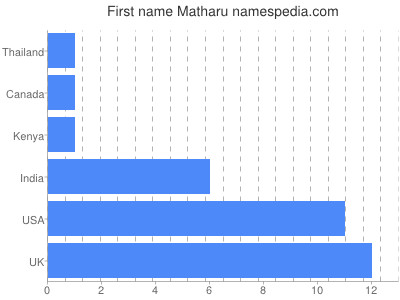Family namesMatharu Parvinder (1)Matharu Mohinder (1)Matharu Rajinder (1)Matharu Reena (1)Matharu Kuldeep (1)Matharu Kamaljit (1)Matharu Harvinder (1)Matharu Charanjit (1)Matharu Jittlar (1)Matharu Harbinder (1)Matharu Harminder (1)Matharu Harpreet (1)Matharu Pushvinder (1)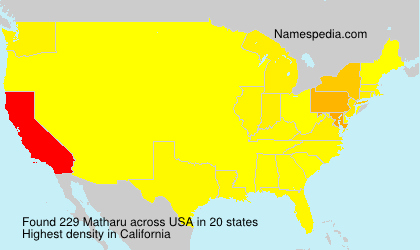Matharu reversed is Urahtam
Name contains 7 letters - 42.86% vowels and 57.14% consonants.

Anagrams: Mtauhra Hatarum Haamurt Mahtuar Atuhram
Misspells: Motharu Mathalu Matharru Matharua Mtaharu Mathaur Mathrau

Rhymes: Amaru Subaru manu onto yahoo fondu argue

Meaning of this name is unknown.

Tarsem Matharu says: Matharus were fierce warriors especially during, the time when the Matharu tribe, had converted to Sikhism; they fought numbers of wars for Guru Gobind Singh, Banda Singh Bahadur and Jassa Singh Ramgarhia. 'Matharu or Matharoo (ਮਠਾੜੂ in Gurmukhī script) is a prominent Sikh clan belonging to the Jatt tribe. Sir Denzil Ibbetson counted the Matharus as one of the major Jatt tribes of the Punjab and the Northwest Frontier Province, centred in Amritsar, Jalandhar, Lahore and Ludhiana. The origin of Matharu was a Jatt clan.Later they were thought of Ramgharia / Tarkhan /Jatt etc. Matharu Jatts are dominant in some villages of Punjab including Jattana, Chounkimaan, Nakodar and areas around Moga. Matharus are widely known as successful landowning farmers through Punjab today. Matharus claiming Jadaun Rajput ancestry are found in Sialkot, Narowal, Gujranwala, Hoshiarpur, Jalandhar, Patiala and Ludhiana districts. Variant forms of the name include Matharoo, Matharu, Matharo, Mathru and Matroo. Matharu’s live in Canada, India, U.K., USA etc. They originate from the regions of Amritsar, Jalandhar, Hoshiarpur, Lahore and Ludhiana in Punjab. The name Matharu appears on one of the marble slabs on the premises of the Harmandir Sahib (Golden Temple) in Amritsar. Matharus built battleships in olden times, and a cannon in the Ranthambore Fort at Rajasthan also bears the name Matharu. Matharus also built weapons for and fought in the armies of Guru Gobind Singh, Prithviraj Chauhan and Akbar. In 1984, the Sikh extremist leader Jarnail Singh Bhindranwale started a Sikh militancy against the Indian Government for creating an independent Sikh state in Punjab to Khalistan. A great number of Matharus and its village of Chaunkiman in Jagroan, Punjab were among the sikhs who supported him. The Punjab Gazetteer says of the Matharus, in a description of the Amritsar District,They possess all the good qualities and martial spirit. Thus in the military atmosphere they have the honour to share up to this day, with their allies, the topmost position among the Brotherhood of Lions.[verified]

This page has been visited from the following countries: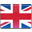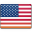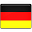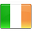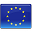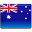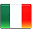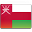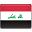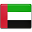Domains ```Matharu.com - REGISTERED Matharu.net - REGISTERED Matharu.co.uk - REGISTERED Matharu.de - FREE ```

Famous people: Kiran Matharu

Writers: M K. Matharu

Recent searches: Kolan Matthee Meya Aggoune Kaats Power Prael Kojiro Brunken Guinart
Top searches: Socrates Sayoc Blumberg Dragos Sithole Davide Blaha Vitelaru Ali Mcshane
Random names: Daoe Parrocho Juhyoung Wizinska Kischkel Kaluri Tereseta Padkowski Melixsa Mervaux

Do you know more about the meaning
or about the origins of this name?